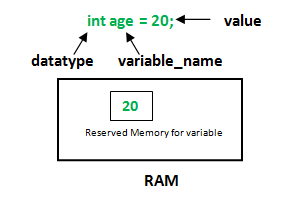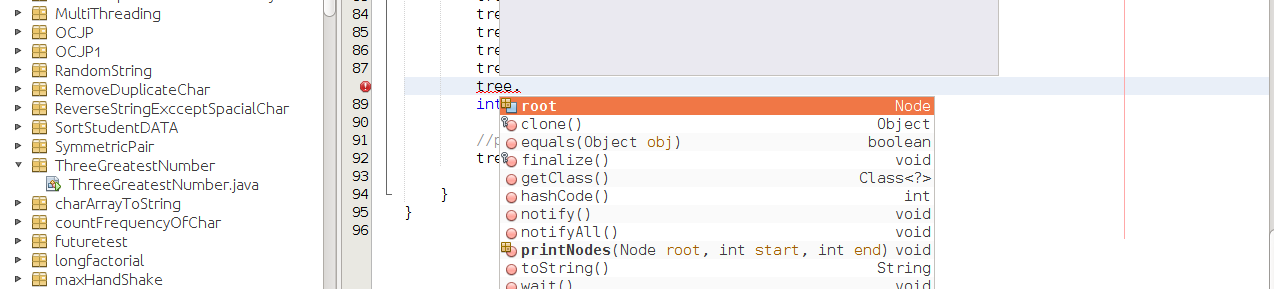Open in App
Not now

# What is the difference between field, variable, attribute, and property in Java

• Difficulty Level : Medium
• Last Updated : 28 Mar, 2022

Variable A variable is the name given to a memory location. It is the basic unit of storage in a program. The value stored in a variable can be changed during program execution. Each variable has a type, such as int, double or Object, and a scope. Class variable may be instance variable, local variable or constant. Also, you should know that some people like to call final non-static variables. In Java, all the variables must be declared before use.Field A data member of a class. Unless specified otherwise, a field can be public, static, not static and final.

## JAVA

 `public` `class` `Customer {` `    ``// Fields of customer``    ``final` `String field1 = "Fixed Value";``    ``int` `name;``}`

Attribute An attribute is another term for a field. It’s typically a public field that can be accessed directly. Let’s see a particular case of Array, the array is actually an object and you are accessing the public constant value that represents the length of the array. In NetBeans or Eclipse, when we type object of a class and after that dot(.) they give some suggestion those suggestion is called Attribute. Note: Here Never Show Private FieldsProperty It is also used for fields, it typically has getter and setter combination. Example:

## JAVA

 `public` `class` `Test {``    ``private` `int` `number;` `    ``public` `int` `getNumber()``    ``{``        ``return` `this``.number;``    ``}` `    ``public` `void` `setNumber(``int` `num)``    ``{``        ``this``.number = num;``    ``}``}`

## JAVA

 `public` `class` `Variables {` `    ``// Constant``    ``public` `final` `static` `String name = "robot";` `    ``// Value``    ``final` `String dontChange = "India";` `    ``// Field``    ``protected` `String river = "GANGA";` `    ``// Property``    ``private` `String age;` `    ``// Still the property``    ``public` `String getAge()``    ``{``        ``return` `this``.age;``    ``}` `    ``// And now the setter``    ``public` `void` `setAge(String age)``    ``{``        ``this``.age = age;``    ``}``}`

My Personal Notes arrow_drop_up